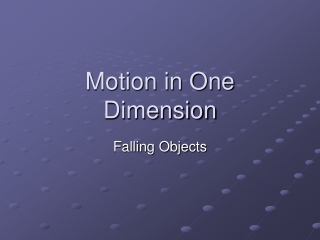DownloadDownload PresentationMotion in One Dimension

# Motion in One Dimension

Télécharger la présentation## Motion in One Dimension

- - - - - - - - - - - - - - - - - - - - - - - - - - - E N D - - - - - - - - - - - - - - - - - - - - - - - - - - -
##### Presentation Transcript

1. Motion in One Dimension Falling Objects

2. Free Fall • Free fall – motion of an object falling with a constant acceleration • Free fall acceleration due to gravity is represented by g • On Earth, g=9.81m/s2 • It is directed toward the center of the Earth • In calculations, g should be negative (-9.81m/s2) • Free fall acceleration is constant • If there is no air resistance, all objects fall at the same rate

3. Free Fall • What goes up must come down • Objects thrown up have to deal with negative free fall acceleration as soon as they are thrown • Eventually, this overcomes the positive acceleration sending them up and returns them to the Earth • At the height of the throw, velocity equals zero for just an instant • Acceleration equals -9.81m/s2

4. Free Fall • When a falling object returns to the height from where it was thrown the velocity is now equal and opposite the initial velocity • Use the kinematic equations from the previous section • a becomes g • ∆x becomes ∆y as we change axis

5. g=-9.81m/s2 Free Fall